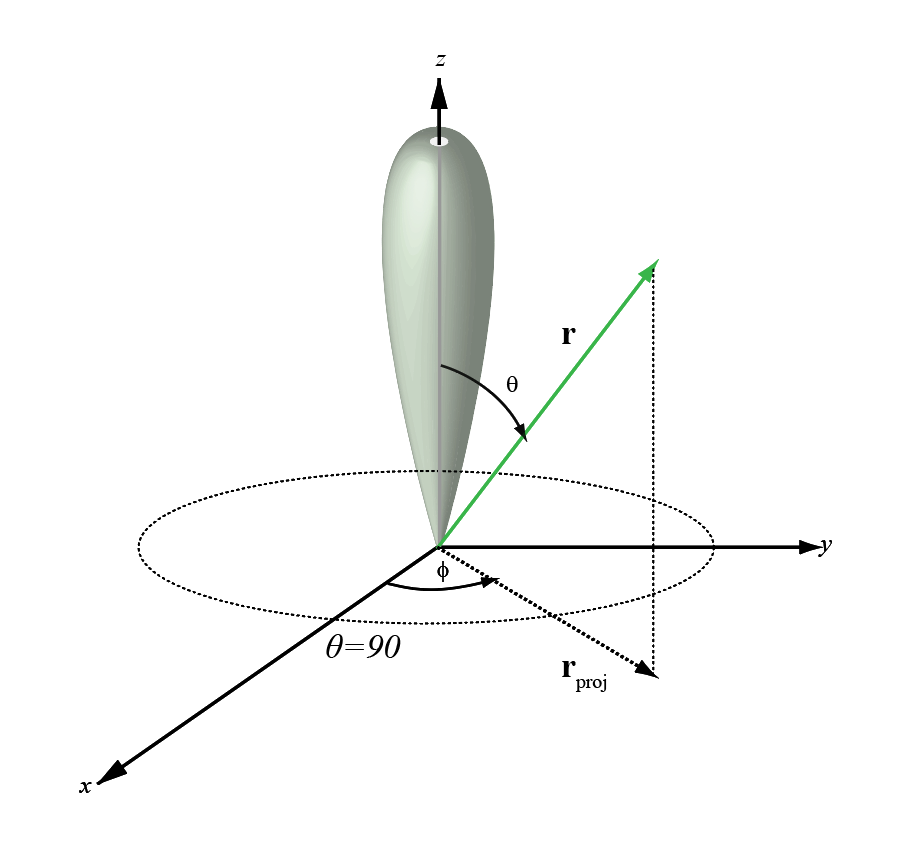# azel2phitheta

Convert angles from azimuth-elevation form to phi-theta form

## Syntax

``PhiTheta = azel2phitheta(AzEl)``
``PhiTheta = azel2phitheta(AzEl,RotAx)``

## Description

example

````PhiTheta = azel2phitheta(AzEl)` converts the azimuth/elevation angle pairs to their corresponding phi/theta angle pairs.```

example

````PhiTheta = azel2phitheta(AzEl,RotAx)` also specifies the choice of phi-theta angle convention using `RotAx`.```

## Examples

collapse all

Find the phi-theta representation for 30° azimuth and 10° elevation for the convention where phi is defined from the y-axis to the z-axis, and theta is defined from the x-axis toward the yz-plane.

`PhiTheta = azel2phitheta([30;10])`
```PhiTheta = 2×1 19.4254 31.4749 ```

Find the phi-theta representation for 30° azimuth and 10° elevation for the convention with phi defined from the x-axis to the y-axis, and theta defined from the z-axis toward the xy-plane.

`PhiTheta = azel2phitheta([30;10],false)`
```PhiTheta = 2×1 30 80 ```

## Input Arguments

collapse all

Azimuth and elevation angles, specified as a two-row matrix. Each column of the matrix represents an angle in degrees, in the form [azimuth; elevation].

Data Types: `double`

Phi-theta angle convention selection, specified as `true` or `false`.

• If `RotAx` is `true`, the phi angle is defined from the y-axis to the z-axis and the theta angle is defined from the x-axis toward the yz-plane.

• If `RotAx` is `false`, the phi angle is defined from the x-axis to the y-axis and the theta angle is defined from the z-axis toward the xy- plane. (see Alternative Definition of Phi and Theta).

Data Types: `double`

## Output Arguments

collapse all

Phi and theta angles, returned as a two-row matrix. Each column of the matrix represents an angle in degrees, in the form [phi; theta]. The matrix dimensions of `PhiTheta` are the same as those of `AzEl`.

collapse all

### Azimuth and Elevation Angles

The azimuth angle of a vector is the angle between the x-axis and the orthogonal projection of the vector onto the xy plane. The angle is positive in going from the x axis toward the y axis. Azimuth angles lie between –180 and 180 degrees. The elevation angle is the angle between the vector and its orthogonal projection onto the xy-plane. The angle is positive when going toward the positive z-axis from the xy plane. By default, the boresight direction of an element or array is aligned with the positive x-axis. The boresight direction is the direction of the main lobe of an element or array.

Note

The elevation angle is sometimes defined in the literature as the angle a vector makes with the positive z-axis. The MATLAB® and Phased Array System Toolbox™ products do not use this definition.

This figure illustrates the azimuth angle and elevation angle for a vector shown as a green solid line.### Phi and Theta Angles

The phi angle (φ) is the angle from the positive y-axis to the vector’s orthogonal projection onto the yz plane. The angle is positive toward the positive z-axis. The phi angle is between 0 and 360 degrees. The theta angle (θ) is the angle from the x-axis to the vector itself. The angle is positive toward the yz plane. The theta angle is between 0 and 180 degrees.

The figure illustrates phi and theta for a vector that appears as a green solid line.The coordinate transformations between φ/θ and az/el are described by the following equations

`$\begin{array}{l}\mathrm{sin}el=\mathrm{sin}\varphi \mathrm{sin}\theta \\ \mathrm{tan}az=\mathrm{cos}\varphi \mathrm{tan}\theta \\ \mathrm{cos}\theta =\mathrm{cos}el\mathrm{cos}az\\ \mathrm{tan}\varphi =\mathrm{tan}el/\mathrm{sin}az\end{array}$`

This transformation applies when `RotAx` is `true`.

### Alternative Definition of Phi and Theta

The phi angle (φ) is the angle from the positive x-axis to the vector’s orthogonal projection onto the xy plane. The angle is positive toward the positive y-axis. The phi angle is between 0 and 360 degrees. The theta angle (θ) is the angle from the z-axis to the vector itself. The angle is positive toward the xy plane. The theta angle is between 0 and 180 degrees.

The figure illustrates φ and θ for a vector that appears as a green solid line.`$\begin{array}{l}\varphi =az\\ \theta =90-el\\ az=\varphi \\ el=90-\theta \end{array}$`

## Version History

Introduced in R2012a